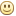Discussion in "General help Guidance and Discussion" started by    Prakruti    May 4, 2008.
Sun May 04 2008, 02:29 am
How to divide a 16 bit data by 8 bit number in 8051 microcontroller????
I want ot write an assembly code in s51, but nor happening. Can any body tell me the logic or the program plz?????

Thank u.
Sun May 04 2008, 03:08 pm
check this file i had downloaded from net. it contains all mathematical routines. modify it according to the routines u require.
Attachment
Prakruti like this.
Tue Oct 06 2009, 09:45 am
a big problem,,,i cant figure out what is r4 and r5 doing in this program because it has not been defined or altered, how can it be taken into accumulator and shifted left and ultimately affecting answer, please help

;====================================================================
; subroutine UDIV16
; 16-Bit / 16-Bit to 16-Bit Quotient & Remainder Unsigned Divide
;
; input: r1, r0 = Dividend X
; r3, r2 = Divisor Y
;
; output: r1, r0 = quotient Q of division Q = X / Y
; r3, r2 = remainder
;
; alters: acc, B, dpl, dph, r4, r5, r6, r7, flags
;====================================================================

UDIV16: mov r7, #0 ; clear partial remainder
mov r6, #0
mov B, #16 ; set loop count

div_loop: clr C ; clear carry flag
mov a, r0 ; shift the highest bit of
rlc a ; the dividend into...
mov r0, a
mov a, r1
rlc a
mov r1, a
mov a, r6 ; ... the lowest bit of the
rlc a ; partial remainder
mov r6, a
mov a, r7
rlc a
mov r7, a
mov a, r6 ; trial subtract divisor
clr C ; from partial remainder
subb a, r2
mov dpl, a
mov a, r7
subb a, r3
mov dph, a
cpl C ; complement external borrow
jnc div_1 ; update partial remainder if
; borrow
mov r7, dph ; update partial remainder
mov r6, dpl
div_1: mov a, r4 ; shift result bit into partial
rlc a ; quotient
mov r4, a
mov a, r5
rlc a
mov r5, a
djnz B, div_loop
mov a, r5 ; put quotient in r0, and r1
mov r1, a
mov a, r4
mov r0, a
mov a, r7 ; get remainder, saved before the
mov r3, a ; last subtraction
mov a, r6
mov r2, a
ret
Tue Oct 06 2009, 04:01 pm
R4, R5 used to calculate quotient in the code. check it.
Wed Oct 07 2009, 02:43 pm
Thanks a lot,,,,that is what comment is tellling but my doubt is

div_1:

mov a, r4 ; shift result bit into partial
rlc a ; quotient
mov r4, a
mov a, r5
rlc a
mov r5, a
djnz B, div_loop
mov a, r5 ; put quotient in r0, and r1
mov r1, a
mov a, r4

here we see that this is the first time r4 comes into light, it has not been previously defined, now we r taking contents of r4 in a because i cannot figure out what is value stored in r4 what will then come in accumulator, and what is being shifted,same is the case with r5 also

my question is what is value in r4 and r5 which is being shifted,because if they arent initialised, they will be 00h whose rotation will be useless

thank you,
regards
tushar
Wed Oct 07 2009, 06:15 pm
hiiiiiiii u go thru this 16 bit divide by 16 bit if u wanna use 16 bit divide by 8 bit then make 00h for higher byte.
Attachment
Thu Oct 08 2009, 04:01 pm
they are assumed to be zero i believe, try it use keil simulator you will get the answerSat May 01 2010, 09:39 am
guys
thanks for the code
but what i found was the answers do not match with the calculator
the calculator gives 0282 as the quotient when dividing 5555h by 22h
and the program posted by sameet gives the answer as 2202 if i use the higher byte to be 00h

kindly advice on the sameWed May 05 2010, 03:19 pm
now I have edited program and u will get the decimal value instead of hex value u can go through it.

e.g 5555H /22H = 246d and 282H
Attachment

### Recent

RandallSwels
Thu Sep 28 2023, 09:25 pm
Zaemlmoche
Tue Sep 26 2023, 06:36 pm
StanleyMek
Tue Sep 26 2023, 01:43 pm
Vikiayf
Tue Sep 26 2023, 05:28 am
Lunteecrenope
Tue Sep 26 2023, 12:51 am
Senyapralk
Mon Sep 25 2023, 08:14 pm
Rosalbazem
Mon Sep 25 2023, 05:55 pm
Robertquorm
Sun Sep 24 2023, 09:57 pm